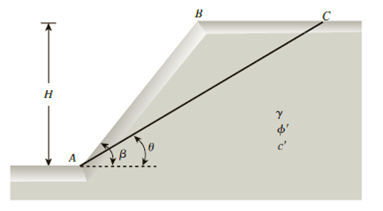Chapter 15, Problem 15.8PPrinciples of Geotechnical Enginee...

9th Edition
Braja M. Das + 1 other
ISBN: 9781305970939

Solutions

Chapter
SectionPrinciples of Geotechnical Enginee...

9th Edition
Braja M. Das + 1 other
ISBN: 9781305970939
Textbook Problem

Refer to Problem 15.7. With all other conditions remaining the same, what would be the factor of safety against sliding for the trial wedge ABC if the height of the slope were 9 m?15.7 Figure 15.49 shows a slope with an inclination of β = 58°. If AC represents a trial failure plane inclined at an angle θ = 32° with the horizontal, determine the factor of safety against sliding for the wedge ABC. Given: H = 6 m; γ = 19 kN/m3, ϕ ′ = 21 ° , and c′ = 38 kN/m2.Figure 15.49

To determine

Find the factor of safety Fs against sliding for the trial wedge ABC if the height of the slope is

9 m.

Explanation

Given information:

The vertical height (H) of the soil is 9.0 m.

The slope with an inclination β is 58°.

The failure plane inclined at an angle θ of 32°.

The angle of friction ϕ is 21°.

The cohesion c is 38kN/m2.

The unit weight γ of the soil is 19kN/m3.

Calculation:

Draw the free body diagram of the wedge ABC as in Figure 1.

Determine the weight W of the wedge ABC using the formula.

W=12γH2[sin(βθ)sinβsinθ]

Substitute 19kN/m3 for γ, 9.0 m for H, 58° for β, and 32° for θ.

W=12(19)(9)2[sin(58°32°)sin58°sin32°]=750.62kN

Determine the normal component Ta using the relation.

Ta=Wsinθ

Substitute 750.62 kN for W and 32° for θ.

Ta=750.62sin32°=397.76kN

Determine the tangential component Na using the relation.

Na=Wcosθ

Substitute 750.62 kN for W and 32° for θ.

Na=750

Still sussing out bartleby?

Check out a sample textbook solution.

See a sample solution

The Solution to Your Study Problems

Bartleby provides explanations to thousands of textbook problems written by our experts, many with advanced degrees!

Get Started

In your own words, explain what is meant by ethics.

Engineering Fundamentals: An Introduction to Engineering (MindTap Course List)

Define each of the following terms: a. data b. field c. record d. file

Database Systems: Design, Implementation, & Management

What tool is used to clean a file?

Precision Machining Technology (MindTap Course List)

Find the rectangular representation of the force F, given that its magnitude is 320 N.

International Edition---engineering Mechanics: Statics, 4th Edition

What angle is the pipe at in the 6G position?

Welding: Principles and Applications (MindTap Course List)